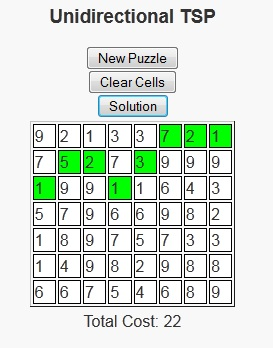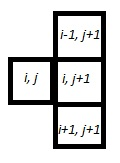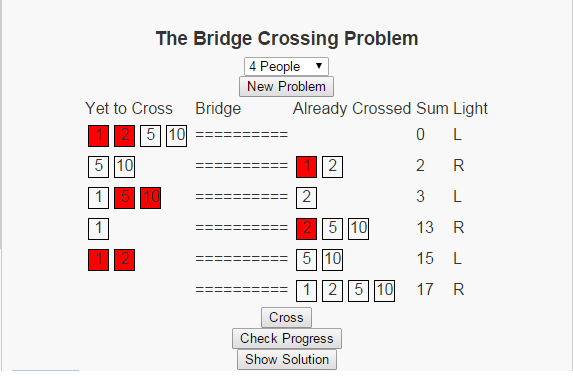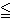# Unidirectional TSP PuzzlesAs we’ve entered the late spring into early summer season, I’ve found myself wanting to go out more to sit and enjoy the weather. One of these days recently I sat in the park with a good book. On this occurrence, I decided not to go with a novel as I had just finished “Incarceron“, “The Archer’s Tale“, and “14 Stones” – all of which were good reads, but I felt like taking a break from the novels.

Just as a side note, 14 Stones is a free book available on smashwords.com and I’ve now read about 6 books from smashwords.com and haven’t been disappointed yet. My favorite is still probably “The Hero’s Chamber” because of the imagery of the book, but there are some well written ebooks available there by some good up and coming writers for a reasonable price, with some being free.

So with the desire to read, but not being in the mood for novels I decided to pick up one of my non-text but still educational books that make me think. This day it was “Programming Challenges“. I browsed through the book until I found one that I could lay back, look at the water, and think about how to solve it.

The programming puzzle the peaked my interest was called “Unidirectional TSP”. We are given a grid with m rows and n columns, with each cell showing the cost of using that cell. The user is allowed to begin in any cell in the first column and is asked to reach any cell in the last column using some minimum cost path. There is an additional constraint that once a cell is selected in a column, a cell in the next column can only be chosen from the row directly above, the same row, or the row directly below. There is a javascript version of this puzzle available here.Fundamentally, the problem is asking for a path of shortest length. Many shortest length problems have a greedy structure, but this one gained my interest because the greedy solution is not always optimal in this case. So I took a moment to figure out the strategy behind these problems. Once I had that solution, I decided that it would be a good program to write up as a puzzle.

In this puzzle version, users will click the cells they wish to travel in each column in which case they will turn green (clicking again will turn them white again). Once the user clicks on a cell in the last column, they will be notified of whether or not they have chosen the minimum path. Or if users are unable to solve a puzzle, the “Solution” button can be pressed to show the optimal path and its cost.

# The Bridge Crossing Problem

Most puzzles are fun in their own right. Some puzzles are so fun that they have the added benefit that they are likely to come up in unexpected places, like maybe in a job interview. I was recently reading a paper by Günter Rote entitled “Crossing the Bridge at Night” where Rote analyzes such a puzzle. Upon finishing the paper, I decided to write a script so that users could see the general form of this puzzle.The problem can be stated as follows: There is a set of people, lets make the set finite by saying that there are exactly n people, who wish to cross a bridge at night. There are a few restrictions that make crossing this bridge somewhat complicated.

• Each person has a travel time across the bridge.
• No more than two people can cross the bridge at one time.
• If two people are on the bridge together, they must travel at the pace of the slower person.
• There is only one flashlight and no party (of one or two people) can travel across the bridge without the flashlight.
• The flashlight cannot be thrown across the bridge, and nobody can go to the store to purchase another flashlight

The image above shows the optimal solution when the 4 people have travel times of 1, 2, 5, and 10. The script I have written allows users to work with different numbers of people with random travel times. Give it a try and see if you can spot the patterns in the solution.

# The Assignment ProblemSuppose you are the owner of a company and need to delegate tasks to your employees. You’ve generated a table that tells how long (in minutes) you think it would take each person to accomplish each individual task (called Jobs). Your goal is to find an assignment of people to jobs that minimizes the total amount of time it will take to complete all jobs. The requirements are that each job must be completed by only one person, and each person can complete only one job.

We can think of the employees at the job as our supply and the tasks as the demand. In order for this problem to have a feasible solution, we must have enough people (supply) to complete the number of jobs (demand). Because of this, our examples will all include situations where there are exactly the same number of people as jobs.

To solve this problem, we must first generate an initial assignment and see how good this assignment is. There are several ways of generating an initial solution, but two that I wanted to focus on are the “NorthWest Corner Rule” and the “Minimum Matrix Method”.

1. The Northwest Corner Rule considers the matrix and repeatedly assigns the top remaining row to the left-most remaining column. If we think of the cost matrix as a being like a map then “top” becomes similar to “north” and left-most becomes similar to “west”, hence the derivation of the name. In assignment problems, this will result in the main diagonal being selected.
2. The Minimum Matrix Method is an iterative method that searches the matrix for the minimum cell in the matrix and assigns that person to the associated job and removes them from consideration and repeats itself until all people have been assigned to jobs.

Once we have formulated an initial feasible solution, we need to check it for optimality. To do this, we use the Network Simplex Method, where we build a basis based on this initial solution. When we consider this problem as a network flow problem, a basis for the problem is a spanning tree (one less than twice the number of nodes in the graph that does not have any cycles) of the network. Because the assignment solution only contains one edge for every two nodes in the graph, we need to add a number of edges to the basis that contains no flow (which makes the solution degenerate) to form this spanning tree.

Once a spanning tree is formulated, we can solve for the dual variables by arbitrarily setting one node’s dual value to zero and solving for the remaining dual variables under the requirement that all arcs in the basis (spanning tree) must satisfy the equation uk + vi = cki for each person k and job i.

When we have dual variables, we can check to see if this solution is optimal by checking to see if all the other constraints are violated. This means that for every person k and every job i, we must have uk + vicki (notice that this is a more relaxed version of what we had when we were solving for the dual variables themselves).

If a constraint is found to be violated, then we need to add the associated edge to the basis and remove an edge on the cycle that is formulated as a result, which generates a new solution.

So check out The Assignment Problem Script and let me know what you think.

# What is a “Hard” Problem?

Throughout our lives, we are introduced to a wide variety of problems. Naturally we tend to think of some problems as more difficult than others. If you were doing the exercises at the end of a chapter in a book, the author generally tends to begin with those exercises that can be completed in a few minutes or directly from the material in the chapter. The exercises in the end tend to be more time consuming and possibly require outside resources. In this sense, these authors tend to compare the difficulty of problems by the amount of time they expect the average reader to take to solve them.

This is a popular way to look at difficulty – in terms of how difficult it is for not only a single person to solve a problem (say you or I), but how long it would take our peers as well as yourself to solve the problem. But how can we measure this? Should I assume that because a problem takes me two years to solve that it would take the average person the same amount of time? Or, if a problem has never been solved, is that because of the difficulty of the problem or could it be for another reason like because no-one had thought of the problem before?

In particular, these questions were being asked in the world of computer science in the 1960s and 1970s. It was around this time that Stephen Cook came up with the concept being able to compare how difficult one problem was in comparison to another. A problem A can be “reduced” to another problem B if a way to solve problem B quickly also gives way to a way to solve problem A quickly.

This concept of reducibility provided a foundation for this concept of difficulty as it was no longer enough to say “I think this problem is hard”, or “the average person would take just as long as me to solve this problem”. No, instead, Cook considered problems that many thought were difficult and showed that the satisfiability problem (the question of whether a given boolean formula contains an assignment to the variables that satisfies all the clauses) could be used to show that all quickly verifiable decision problems (also called problems in NP), where the instances with a “yes” answer have short proofs of the fact that the answer is indeed “yes” could be reduced to this problem.

This meant that the satisfiability problem was at least as hard as every one of these quickly verifiable decision problems, so if this problem could be solved quickly, then every one of these quickly verifiable decision problems could also be solved quickly. So the satisfiability problem is at least as hard as every problem in this class, NP. We call such problems that have this property NP-Hard. Decision Problems that are NP-Hard are called NP-Complete. Richard Karp later published a paper that proposed 21 NP-Complete problems, one of which was the Knapsack Problem I spoke about last time.This concept of NP-Complete gives light to the image that became famous in the book by Garey and Johnson “Computers and Intractability: A Guide to the Theory of NP-Completeness”. It shows that the concept of difficulty still builds on the ability of our peers to solve a given problem. But by introducing a class of “hard” problems, and a litmus test for inclusion into that class, researchers could now consider new problems and determine their difficulty by comparing it to known hard problems.

(a quick note. I’ve used the term “quickly” here, but the formal phrase that I mean by that is that the problem is solvable by an algorithm whose worse case running time is bounded by a polynomial on the input parameters of the problem)

Other Blogs that have covered this topic:
Explorations
To Imaging, and Beyond!

# Learn Duality in Linear ProgrammingI have just completed a script to help learn about duality in linear programming.

Many real world problems can be formulated as Linear Programming problems. There are often many different ways to formulate a single problem. Some of these alternative formulations can easily be proven to be equal via simple algebra and arithmetic. For example, one person may see a problem as maximizing profit while another may see the same problem as minimizing losses. The relationship between the two alternative formulations can then be shown to be that one simply has the negative objective function value of the other.

Sometimes, though, this relationship between alternative formulations is not as easy to detect. Two alternative formulations that arise regularly in linear programming problems are a primal problem and a dual problem. The dual of a linear programming problem is the problem of finding the best bound on the objective function in terms of the constraints. This dual is formulated so that every feasible solution to the dual is a bound on the primal objective function. The Weak Duality Theorem for Linear Programming says that the optimal solution for a minimization problem is always an upper bound for its dual (which is a corresponding maximization problem). Correspondingly, the optimal solution for a maximization problem is always a lower bound for its dual. This leads to the Strong Duality Theorem for Linear Programming which says that if we can find feasible solutions to the primal and dual problems with matching objective function values, then both of these solutions must be optimal for their respective problems.

This shows an importance of duality. What my script provides is a means of formulating the dual of a given problem to help understand the concept.

The rules for constructing a dual linear program are as follows:

 Primal: Dual: The ith constraint is [<=] Variable yi is [>=] 0 The ith constraint is [>=] Variable yi is is [<=] 0 The ith constraint is = Variable yi is unbounded Variable xj is [>=] 0 The jth constraint is [>=] Variable xj is [<=] 0 The jth constraint is [<=] Variable xj is unbounded The jth constraint is =

Other Blogs that have covered this topic:
A Narrow Margin
Optimization and data mining

# Hello, World!

The ideas for this site have been bouncing around inside my head and on my computer for years, and this site is an acknowledgement that it was time to finally act on these ideas.

The site will feature a collection of scripts I have written to help illustrate different concepts. A large part of this will be a flash cards section which will provide an avenue to study or to refresh one’s memory on various subjects. I recognize, though, that all subjects are not easily understood through flash cards and so I also have an examples section where various algorithms are implemented on example problems (problem sets) to provide users with a more hands on experience.

The site will be updated regularly, generally with either new subject areas added to the flash cards database, new scripts added to the examples. I leave open the possibility, though, of entirely new sections being introduced as ideas continue to develop and the site continues to grow.

EDIT:
There are several areas that I would like to take the site, but with each area I have the problems of (a) showing the concept, (b) visualizing the concept, c) showing the intuition behind the concept. Sometimes, I will think of (what I call) more clever ways to teach or learn an idea I’ve already discussed. This may lead to more than one page on the same concept. I encourage you to try all such pages to see if you like any of them.

I hope you enjoy.